by Jeon Lee on January 10, 2019

### Data format and preparation

The data set mtcars is used in the examples below:

``````library(ggplot2)
data(mtcars)
mtcars\$cyl <- as.factor(mtcars\$cyl)
``````                     wt  mpg cyl
Mazda RX4         2.620 21.0   6
Mazda RX4 Wag     2.875 21.0   6
Datsun 710        2.320 22.8   4
Hornet 4 Drive    3.215 21.4   6
Valiant           3.460 18.1   6``````

### ggplot(): build plots piece by piece

As mentioned above, there are two main functions in ggplot2 package for generating graphics:

• The quick and easy-to-use function: qplot()
• The more powerful and flexible function to build plots piece by piece: ggplot()

This section describes briefly how to use the function ggplot(). Recall that, the concept of ggplot divides a plot into three different fundamental parts: plot = data + Aesthetics + geometry.

• data: a data frame.
• Aesthetics: used to specify x and y variables, color, size, shape, ..
• Geometry: the type of plots (histogram, boxplot, line, density, dotplot, bar, .)

### Ex.1: Basic scatter plot

``````# Basic scatter plot
ggplot(data = mtcars, aes(x = wt, y = mpg)) +
geom_point()``````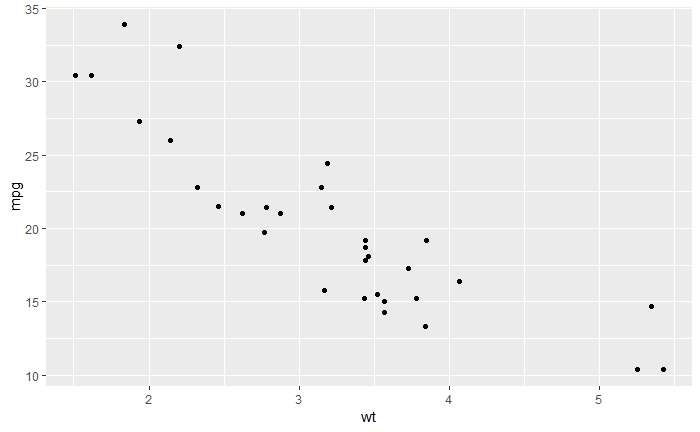``````# Change the point size, and shape
ggplot(mtcars, aes(x = wt, y = mpg)) +
geom_point(size = 2, shape = 23)``````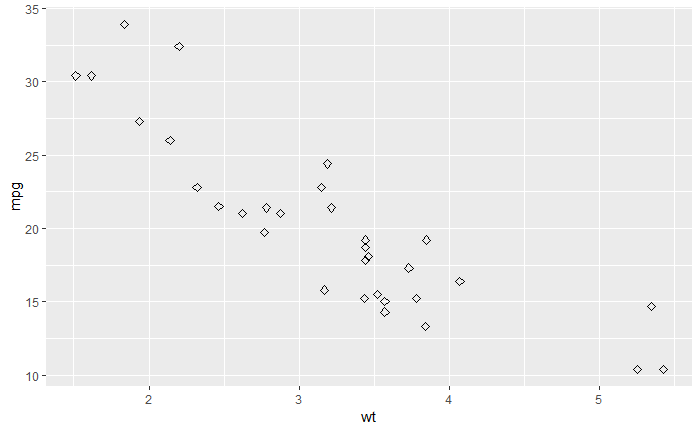### Ex.2: Adding layers to a scatter plot

Possible layers include:

• geom_point() for scatter plot
• geom_smooth() for adding smoothed line such as regression line
• geom_quantile() for adding quantile lines
• geom_rug() for adding a marginal rug
• geom_jitter() for avoiding overplotting
• geom_text() for adding textual annotations

#### a. geom_point(): Scatter plot

``````b <- ggplot(mtcars, aes(x = wt, y = mpg))
# Basic plot
b + geom_point()````````````
# change the color and the point
# by the levels of cyl variable
b + geom_point(aes(color = cyl, shape = cyl)) ``````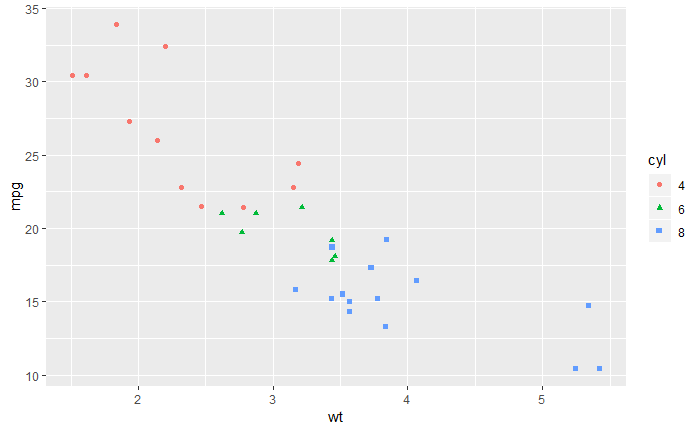``````# Change color manually
b + geom_point(aes(color = cyl, shape = cyl)) +
scale_color_manual(values = c("#999999", "#E69F00", "#56B4E9"))+
theme_minimal()``````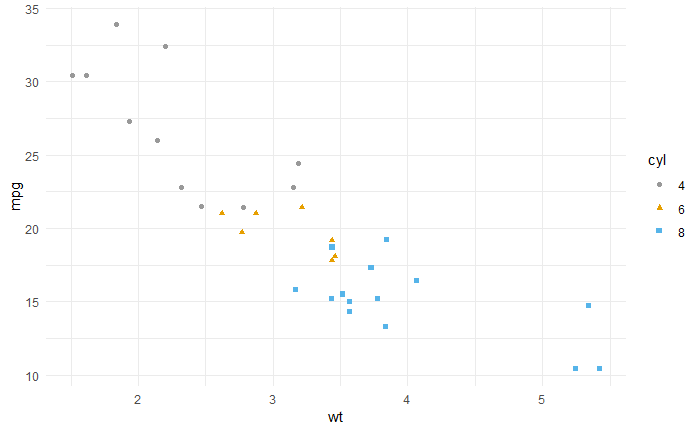#### b. geom_smooth(): Add regression line or smoothed conditional mean

To add a regression line on a scatter plot, the function geom_smooth() is used in combination with the argument method = lm. lm stands for linear model.

``````# Regression line only
b + geom_smooth(method = lm)``````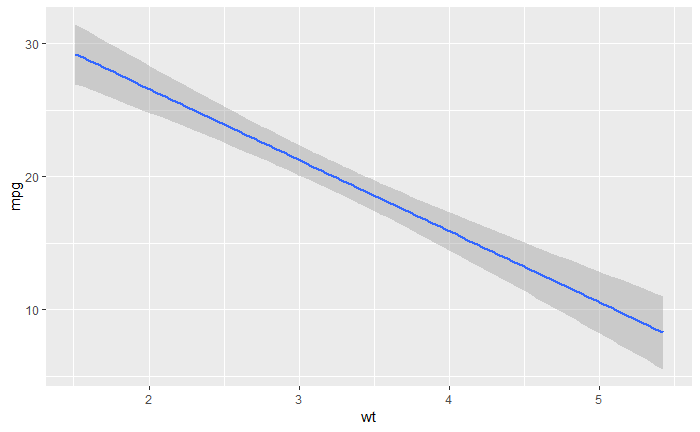``````
# Point + regression line
# Remove the confidence interval
b + geom_point() +
geom_smooth(method = lm, se = FALSE)``````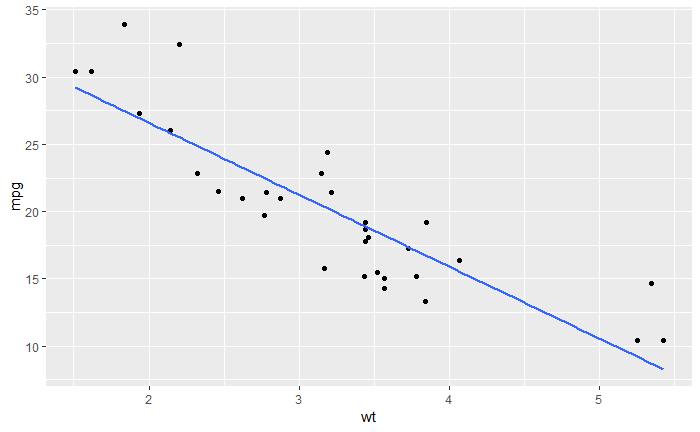``````# loess method: local regression fitting
b + geom_point() + geom_smooth()``````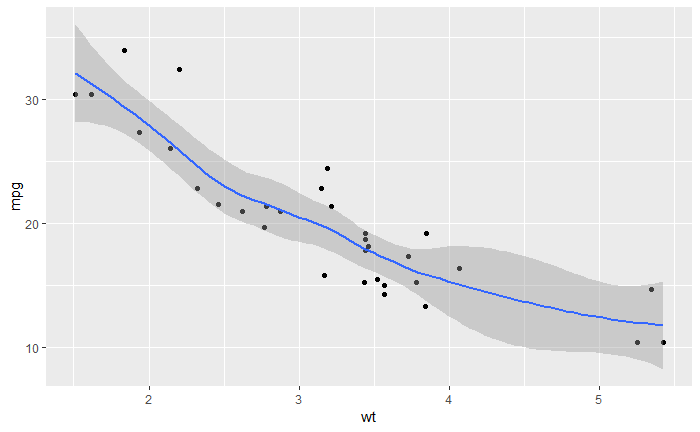``````# Change color and shape by groups (cyl)
b + geom_point(aes(color=cyl, shape=cyl)) +
geom_smooth(aes(color=cyl, shape=cyl),
method=lm, se=FALSE, fullrange=TRUE)``````
``Ignoring unknown aesthetics: shape``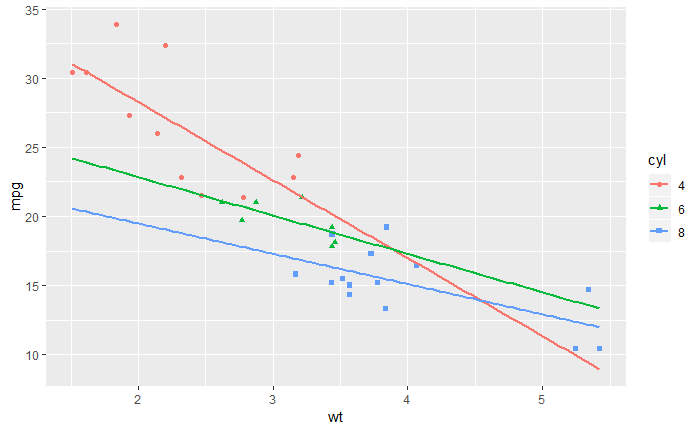#### c. geom_jitter(): Jitter points to reduce overplotting

``````p <- ggplot(mpg, aes(displ, hwy))
# Default scatter plot
p + geom_point()``````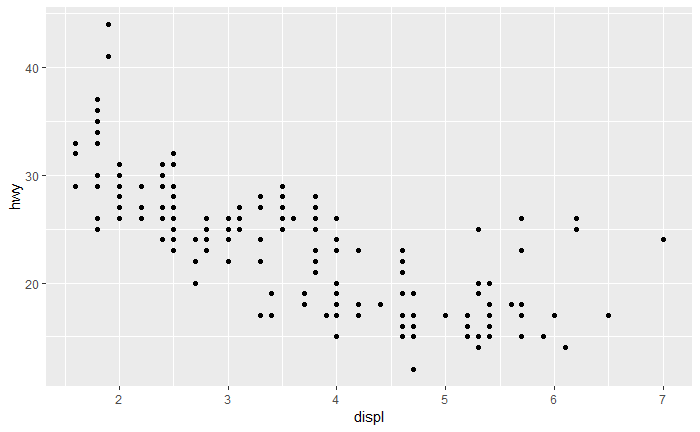``````# Use jitter to reduce overplotting
p + geom_jitter(
position = position_jitter(width = 0.5, height = 0.5))``````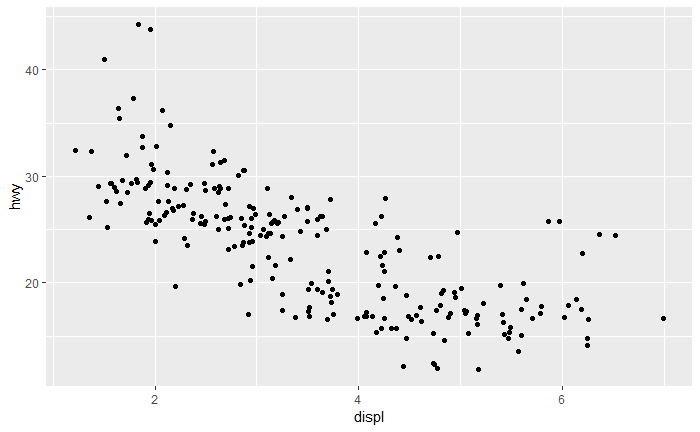#### d. geom_text(): Textual annotations

The argument label is used to specify a vector of labels for point annotations.

``b + geom_text(aes(label = rownames(mtcars)))``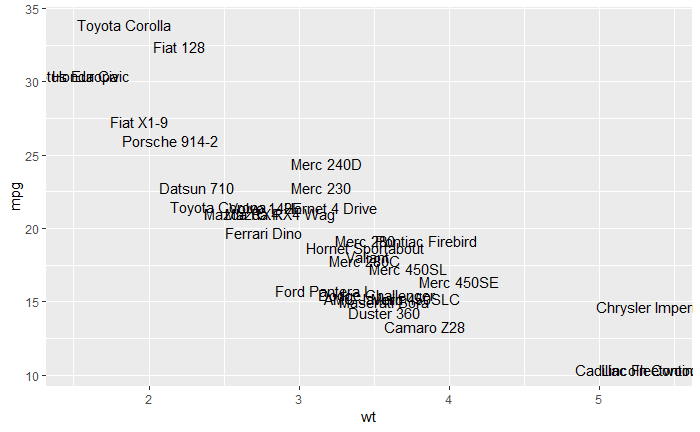``````# colored text
p <- ggplot(mtcars, aes(x=wt, y=mpg, label=rownames(mtcars)))+
geom_point()
p````````````# Color by groups
p + geom_text(aes(color=factor(cyl)))``````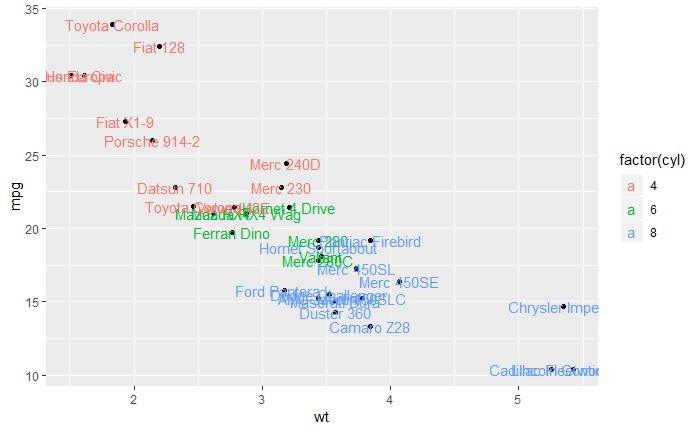### Ex.4: One variable plots

Possible layers are:

• For one continuous variable:
• geom_area() for area plot
• geom_density() for density plot
• geom_dotplot() for dot plot
• geom_freqpoly() for frequency polygon
• geom_histogram() for histogram plot
• stat_ecdf() for empirical cumulative density function
• stat_qq() for quantile - quantile plot
• For one discrete variable:
• geom_bar() for bar plot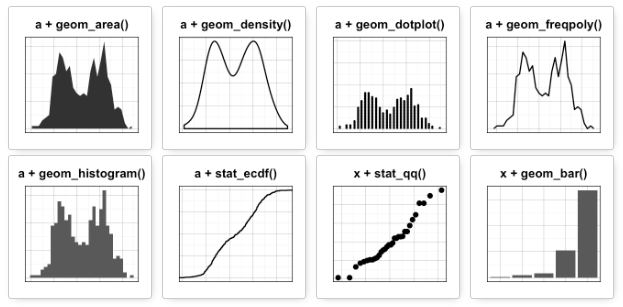#### a. geom_area(): Create an area plot

Manually generate wdata by typing in wdata = data.frame(sex = factor(rep(c(“F”, “M”), each=200)), weight = c(rnorm(200, 55), rnorm(200, 58)))

``````set.seed(1234)
wdata = data.frame(
sex = factor(rep(c("F", "M"), each=200)),
weight = c(rnorm(200, 55), rnorm(200, 58)))
``````  sex   weight
1   F 53.79293
2   F 55.27743
3   F 56.08444
4   F 52.65430``````
``````a <- ggplot(wdata, aes(x = weight))
# Basic plot
a + geom_area(stat = "bin")``````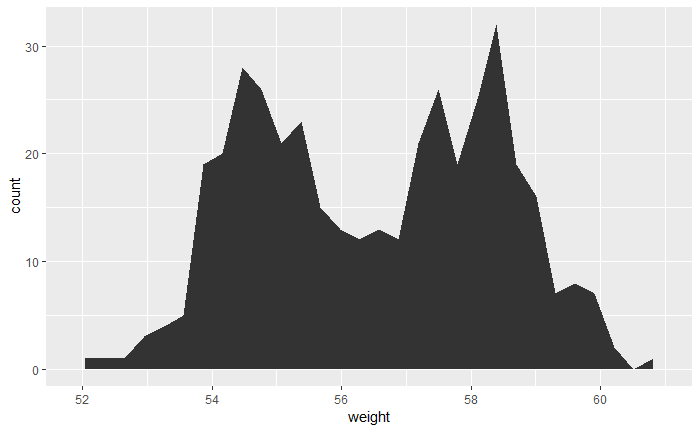``````# change fill colors by sex
a + geom_area(aes(fill = sex), stat ="bin", alpha=0.6) +
theme_classic()``````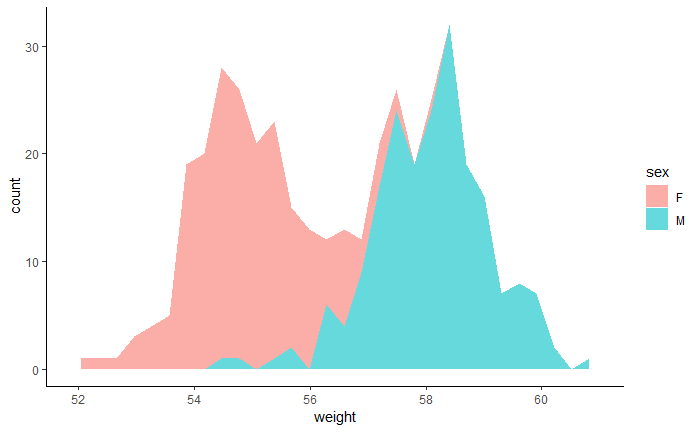#### b. geom_density(): Create a smooth density estimate

geom_density() to create a density plot geom_vline() to add a vertical lines corresponding to group mean values scale_color_manual() to change the color manually by groups

``````# Basic plot
p + geom_density()``````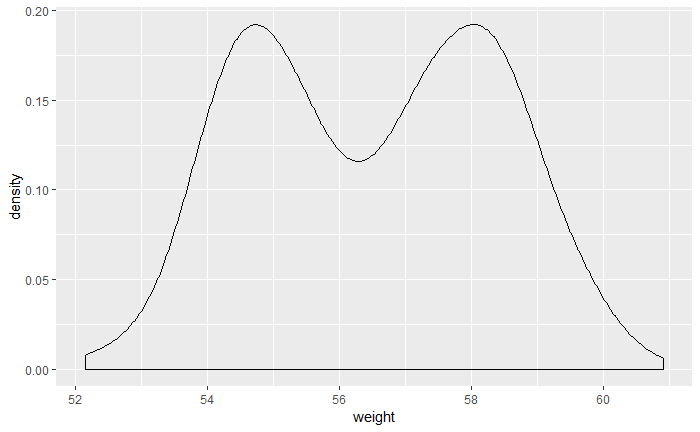``````# change line colors by sex
p + geom_density(aes(color = sex)) ``````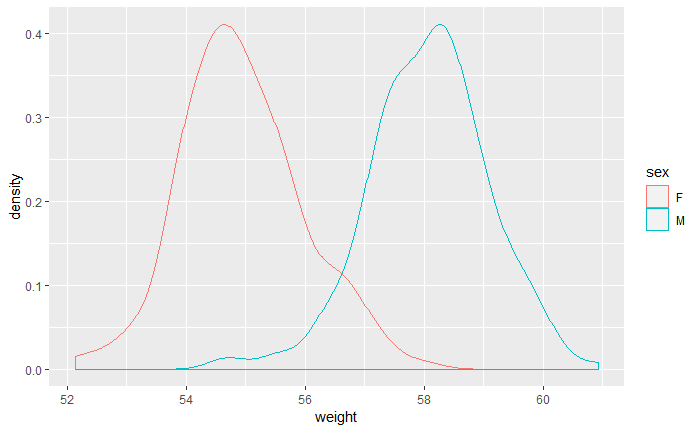``````# Change fill color by sex
# Use semi-transparent fill: alpha = 0.4
p + geom_density(aes(fill = sex), alpha=0.4)``````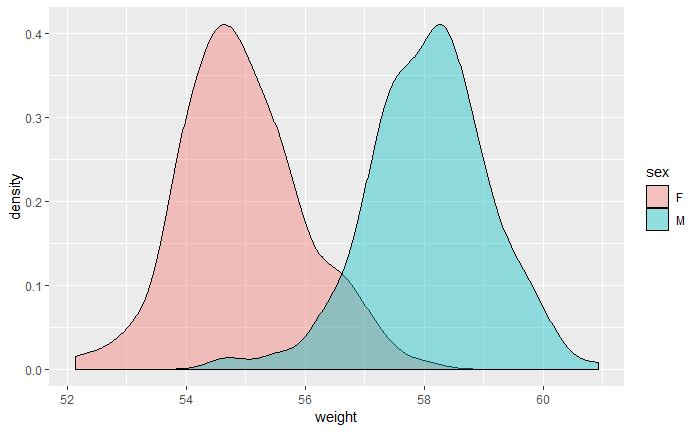#### c. geom_histogram(): Histogram

``````# Basic plot
p + geom_histogram()``````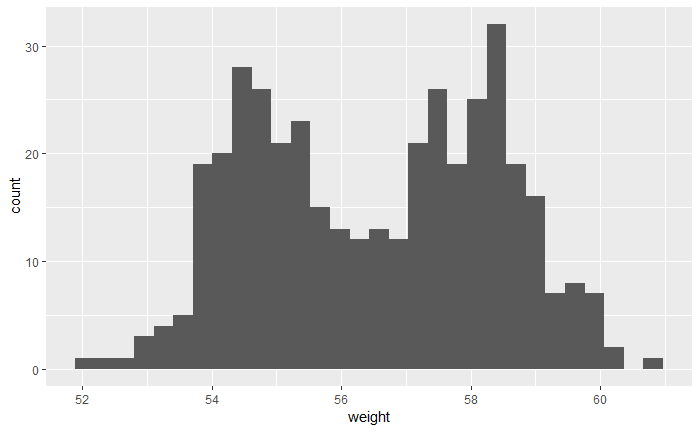``````# change line colors by sex
p + geom_histogram(aes(color = sex), fill = "white", position = "dodge")``````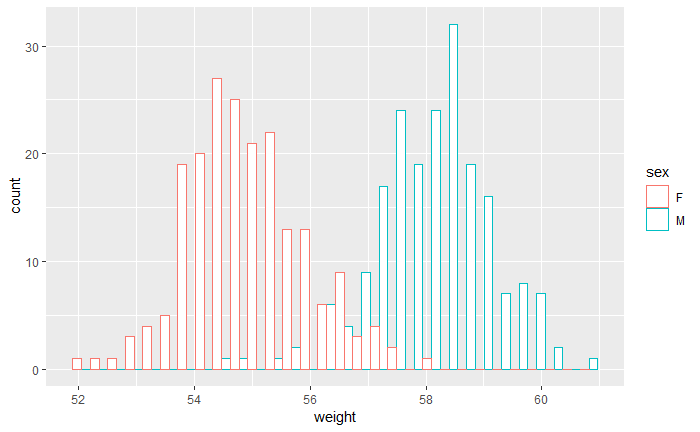#### d. One variable: Discrete

The function geom_bar() can be used to visualize one discrete variable. In this case, the count of each level is plotted. Please use the mpg data set [in ggplot2 package]. The R code is as follow:

``````data(mpg)
b <- ggplot(mpg, aes(fl))
# Basic plot
b + geom_bar()``````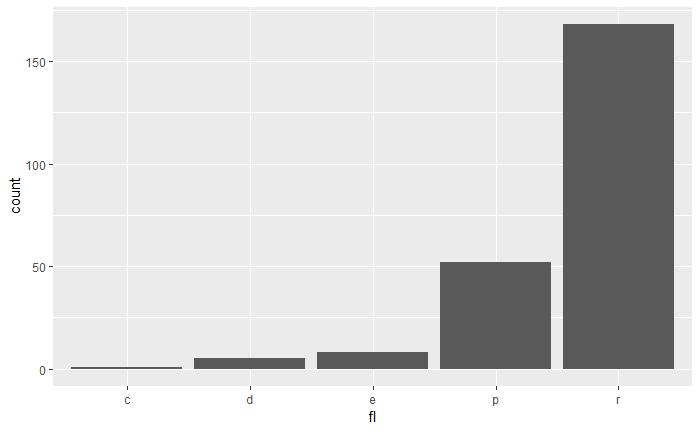``````# Change fill color
b + geom_bar(fill = "steelblue", color ="steelblue") +
theme_minimal()``````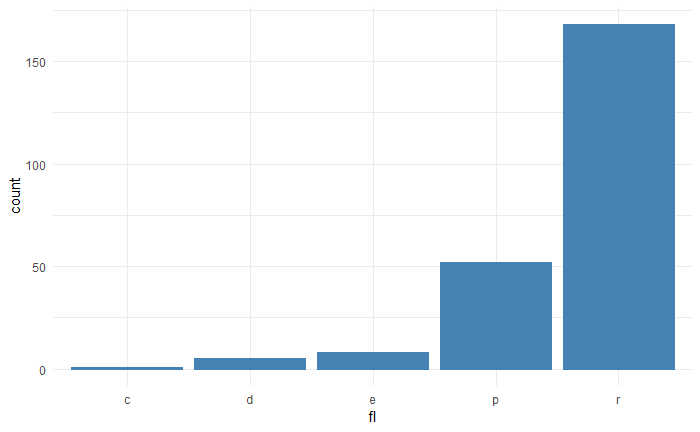### Ex.5: Box plots

Please use the ToothGrowth data set which consists of the continuous variable len (for tooth length) by the discrete variable dose.

``````data("ToothGrowth")
ToothGrowth\$dose <- as.factor(ToothGrowth\$dose)
``````   len supp dose
1  4.2   VC  0.5
2 11.5   VC  0.5
3  7.3   VC  0.5
4  5.8   VC  0.5
5  6.4   VC  0.5
6 10.0   VC  0.5``````
``p <- ggplot(ToothGrowth, aes(x = dose, y = len))``

Possible layers include:

• geom_boxplot() for box plot
• geom_violin() for violin plot
• geom_dotplot() for dot plot
• geom_jitter() for stripchart
• geom_line() for line plot
• geom_bar() for bar plot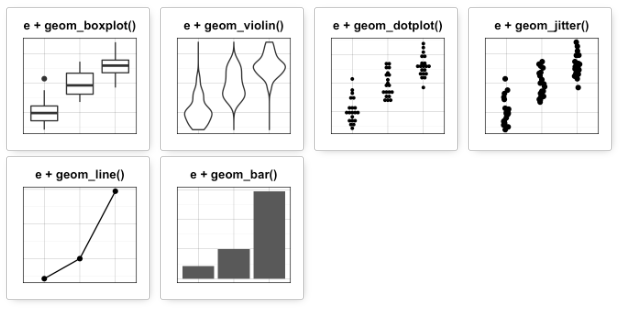#### a. geom_boxplot(): Box and whiskers plot

``````# Default plot
p + geom_boxplot()``````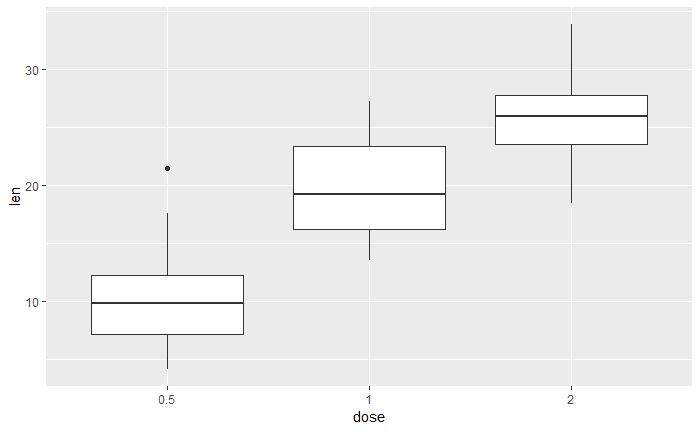``````# Notched box plot
p + geom_boxplot(notch = TRUE)``````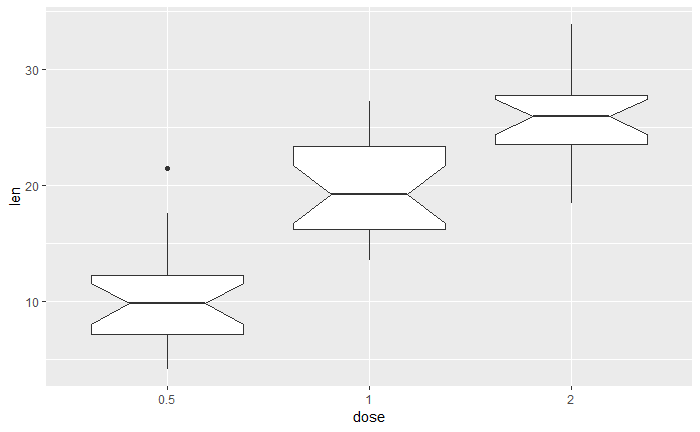``````# Color by group (dose)
p + geom_boxplot(aes(color = dose))``````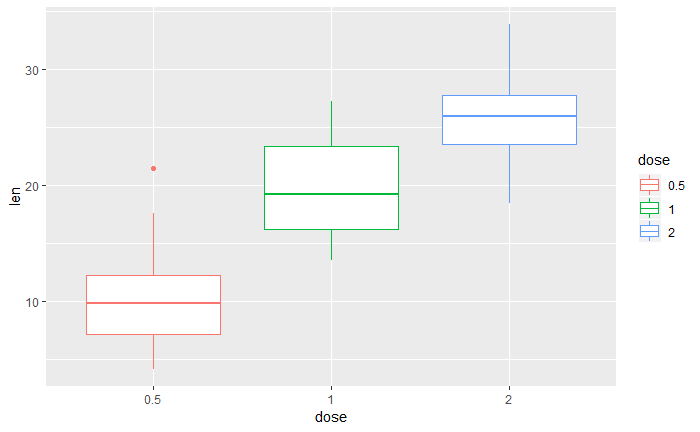``````# Change fill color by group (dose)
p + geom_boxplot(aes(fill = dose))``````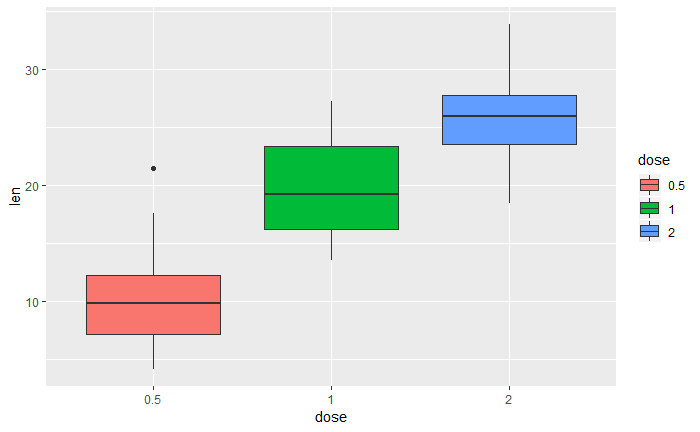``````# Box plot with multiple groups
ggplot(ToothGrowth, aes(x=dose, y=len, fill=supp)) + geom_boxplot()``````